# FrictionPage 1

#### WATCH ALL SLIDES

Slide 1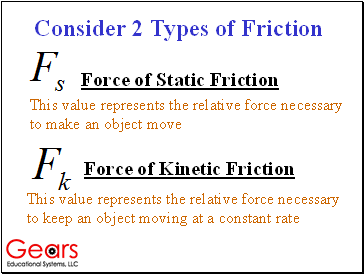## Types of Friction

Force of Kinetic Friction

This value represents the relative force necessary to keep an object moving at a constant rate

Force of Static Friction

This value represents the relative force necessary to make an object move

Slide 2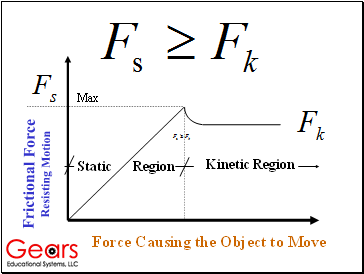Frictional Force Resisting Motion

Force Causing the Object to Move

Kinetic Region

Static Region

Max

Slide 3Frictional Forces Occur When Materials are in Contact

W

fs

F

N

Surfaces in Contact

M1

Spring Scale

F = Force Causing Motion (Pull on Scale)

Fs = Force of Static Friction (Resists Motion)

N = Force Normal Holds Surfaces in Contact

W = Weight of Object ( Mass x Gravity)

Slide 4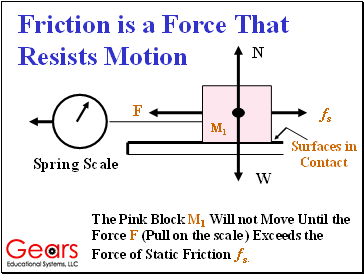Friction is a Force That Resists Motion

W

fs

F

N

Surfaces in Contact

The Pink Block M1 Will not Move Until the Force F (Pull on the scale ) Exceeds the Force of Static Friction fs.

M1

Spring Scale

Slide 5The Relative Force of Static Friction Between 2 Objects is Expressed as the Quotient of the Force (F) Required to Move the Object

W

fs

F

N

Surfaces in Contact

M1

Spring Scale

Divided by the Weight W of the Object

This is Called the Coefficient of Friction

Slide 6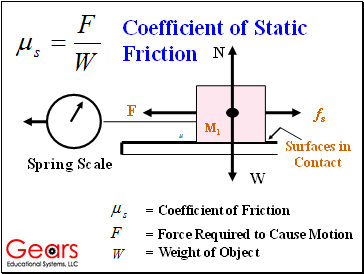W

fs

F

N

Surfaces in Contact

M1

Spring Scale

= Force Required to Cause Motion

= Weight of Object

= Coefficient of Friction

Coefficient of Static Friction

Slide 7Using the Gears-IDS Battery to Calculate The Static

Coefficient of Friction

Slide 8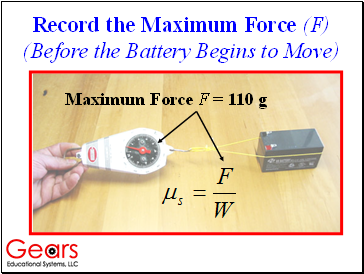Record the Maximum Force (F) (Before the Battery Begins to Move)

Maximum Force F = 110 g

Slide 9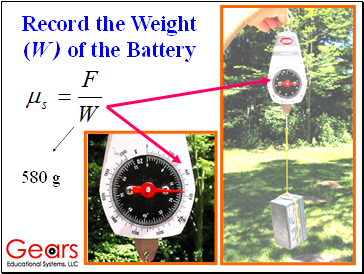Record the Weight (W ) of the Battery

580 g

Slide 10The Coefficient of Static Friction Between the Wood Desktop and the Plastic Battery is Described Algebraically:

= 580 g

= 110 g

= .190

Slide 11The Coefficient of Kinetic Friction Can be Found Using the Same Technique

Go to page:
1  2